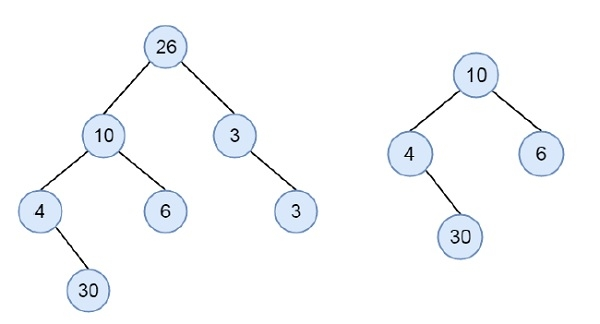# Check if a binary tree is subtree of another binary tree in C++

C++Server Side ProgrammingProgramming

Suppose we have two binary trees. We have to check whether the smaller tree is a subtree of another binary tree or not. Consider these two trees are given.There are two trees. The second tree is the subtree of the first one. To check this property, we will traverse the tree in post-order fashion, then if the subtree rooted with this node is identical to the second tree, then it is subtree.

## Example

Live Demo

#include <bits/stdc++.h>
using namespace std;
class node {
public:
int data;
node *left, *right;
};
bool areTwoTreeSame(node * t1, node *t2) {
if (t1 == NULL && t2 == NULL)
return true;
if (t1 == NULL || t2 == NULL)
return false;
return (t1->data == t2->data && areTwoTreeSame(t1->left, t2->left) && areTwoTreeSame(t1->right, t2->right) );
}
bool isSubtree(node *tree, node *sub_tree) {
if (sub_tree == NULL)
return true;
if (tree == NULL)
return false;
if (areTwoTreeSame(tree, sub_tree))
return true;
return isSubtree(tree->left, sub_tree) || isSubtree(tree->right, sub_tree);
}
node* getNode(int data) {
node* newNode = new node();
newNode->data = data;
newNode->left = newNode->right = NULL;
return newNode;
}
int main() {
node *real_tree = getNode(26);
real_tree->right = getNode(3);
real_tree->right->right = getNode(3);
real_tree->left = getNode(10);
real_tree->left->left = getNode(4);
real_tree->left->left->right = getNode(30);
real_tree->left->right = getNode(6);
node *sub_tree = getNode(10);
sub_tree->right = getNode(6);
sub_tree->left = getNode(4);
sub_tree->left->right = getNode(30);
if (isSubtree(real_tree, sub_tree))
cout << "Second tree is subtree of the first tree";
else
cout << "Second tree is not a subtree of the first tree";
}

## Output

Second tree is subtree of the first tree